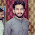# THE VOLUME OF TRIANGULAR PRISM AND ITS CROSS SECTION AREA

The diagram shows a solid in the shape of a triangular prism.
AC = 5 cm, BC = 4 cm and CD = 7 cm.
Angle ABC = 90°.

Mathematics Cambridge IGCSE Test (0580 m16 qp 32)

(a) What does the word prism tell you about the solid in the diagram?
Constant or uniform cross-sectional area. It means if you cut parallel to the base side, it will be
same area with area of the base. The diagram shown below, red area will same with blue area.

(b) Show that AB = 3 cm.
By using Phytagoras Formula, 52 = 42 + 32
It shown that AB = 3 cm.

(c) Calculate the volume of the prism.
volume of the prism formula is Base Area x Height Triangular Prism
= Area of Triangle ABC x Height of Triangular Prism
= ½  x 4 x 3 x 7
= 42 cm squares.

### 2 Komentar di Postingan "THE VOLUME OF TRIANGULAR PRISM AND ITS CROSS SECTION AREA"

1.2.Untuk menyisipkan kode,gunakan tag
[code]KODE ANDA[/code]
Untuk menyisipkan quote,gunakan tag
[blockquote]KATA-KATA ANDA[/blockquote]
Untuk menyisipkan gambar,gunakan tag
[img]URL GAMBAR ANDA[/img]
Untuk menyisipkan video,gunakan tag